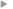## Stability of Constant and Variable Coefficient Semi-Implicit Schemes for the Fully Elastic System of Euler Equations in the Case of Steep Slopes

#### Burgot, Thomas ; Auger, Ludovic ; Bénard, Pierre

Année de publication
2023
Résumé
<p align=justify>Crank-Nicolson temporal schemes are usually employed in most semi-implicit models commonly applied in numerical weather prediction. These schemes are approached by fixed points methods like the iterative centered implicit scheme, where a linear operator is introduced to invert the implicit problem. When this operator is spatially dependent the scheme is a variable coefficient one, and a constant coefficient one otherwise. Constant and variable coefficient temporal schemes are analyzed and tested on idealized test cases to improve numerical stability on the steepest slopes of the orography encountered at hectometric scales. These analyses and experiments are conducted for the fully elastic system of Euler equations for different slopes and different thermal residuals. Because of their low computational cost, several strategies can be easily tested. None of the strategies considered for constant coefficient schemes significantly improves numerical stability without worsening efficiency or accuracy. Hence, constant coefficient schemes are probably not the most suitable schemes for high-resolution computing. A successful strategy consists in using the same features of constant coefficient schemes except for the orographic terms that are implicitly treated, resulting in a variable coefficient scheme. In this case, slopes up to 70° can be easily reached, even in the case of a moderate thermal residual. Since estimates on the condition number of the implicit problem containing orographic terms remains low even in case of steep slopes, the implicit problem should be easily inverted by an iterative solver.</p>
Texte intégralAccès à la notice sur le site du portail documentaire de Météo-FranceListe complète des notices publiques